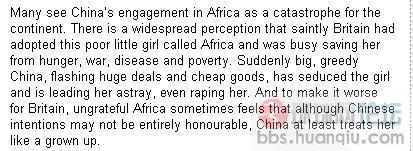# 一點浩然氣,千裏快哉風.## 英媒: 英國紳士收養了非洲, 中國大漢勾引奸汙非洲

(2009-11-12 08:25:36) 下一個“很多人視中國插手非洲事務為非洲大陸的災難。有一種廣為流傳的觀念是，聖潔的英國紳士收養了非洲這個窮苦的小姑娘，拯救她脫離饑餓、戰爭、疾病和貧困。突然間，一個壯實而又貪婪的中國大漢進來插了一腳，用大買賣和便宜貨勾引這個小姑娘，甚至要最終奸汙她。而對於英國紳士來說，更另他們難以接受的是：非洲這個小姑娘對他的養育之恩一點也不領情，反而覺得雖然中國大漢對她的意圖也並不高尚，但至少他把她當成年人看了。”
[ 打印 ]where is the original words?where is the original words?where is the original words?where is the original words?where is the original words?where is the original words?where is the original words?where is the original words?where is the original words?where is the original words?where is the original words?where is the original words?where is the original words?where is the original words?where is the original words?where is the original words?where is the original words?where is the original words?